## Linear Equations: Solutions Using Elimination with Three Variables

Systems of equations with three variables are only slightly more complicated to solve than those with two variables. The two most straightforward methods of solving these types of equations are by elimination and by using 3 × 3 matrices.

To use elimination to solve a system of three equations with three variables, follow this procedure:

1. Write all the equations in standard form cleared of decimals or fractions.

2. Choose a variable to eliminate; then choose any two of the three equations and eliminate the chosen variable.

3. Select a different set of two equations and eliminate the same variable as in Step 2.

4. Solve the two equations from steps 2 and 3 for the two variables they contain.

5. Substitute the answers from Step 4 into any equation involving the remaining variable.

6. Check the solution with all three original equations.

##### Example 1

Solve this system of equations using elimination.All the equations are already in the required form.

Choose a variable to eliminate, say x, and select two equations with which to eliminate it, say equations (1) and (2).Select a different set of two equations, say equations (2) and (3), and eliminate the same variable.Solve the system created by equations (4) and (5).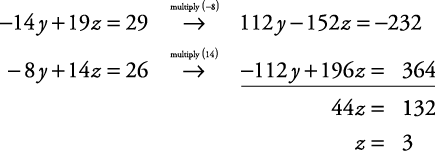Now, substitute z = 3 into equation (4) to find y.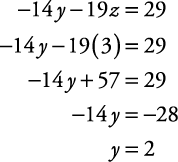Use the answers from Step 4 and substitute into any equation involving the remaining variable.

Using equation (2),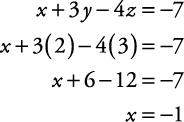Check the solution in all three original equations.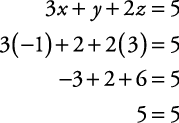The solution is x = –1, y = 2, z = 3.

##### Example 2

Solve this system of equations using the elimination method.Write all equations in standard form.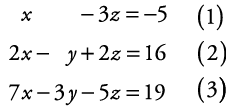Notice that equation (1) already has the y eliminated. Therefore, use equations (2) and (3) to eliminate y. Then use this result, together with equation (1), to solve for x and z. Use these results and substitute into either equation (2) or (3) to find y.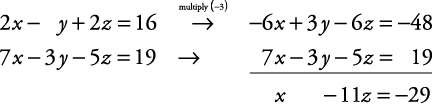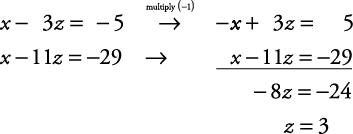Substitute z = 3 into equation (1).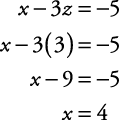Substitute x = 4 and z = 3 into equation (2).Use the original equations to check the solution (the check is left to you).

The solution is x = 4, y = –2, z = 3.

Back to Top
A18ACD436D5A3997E3DA2573E3FD792A